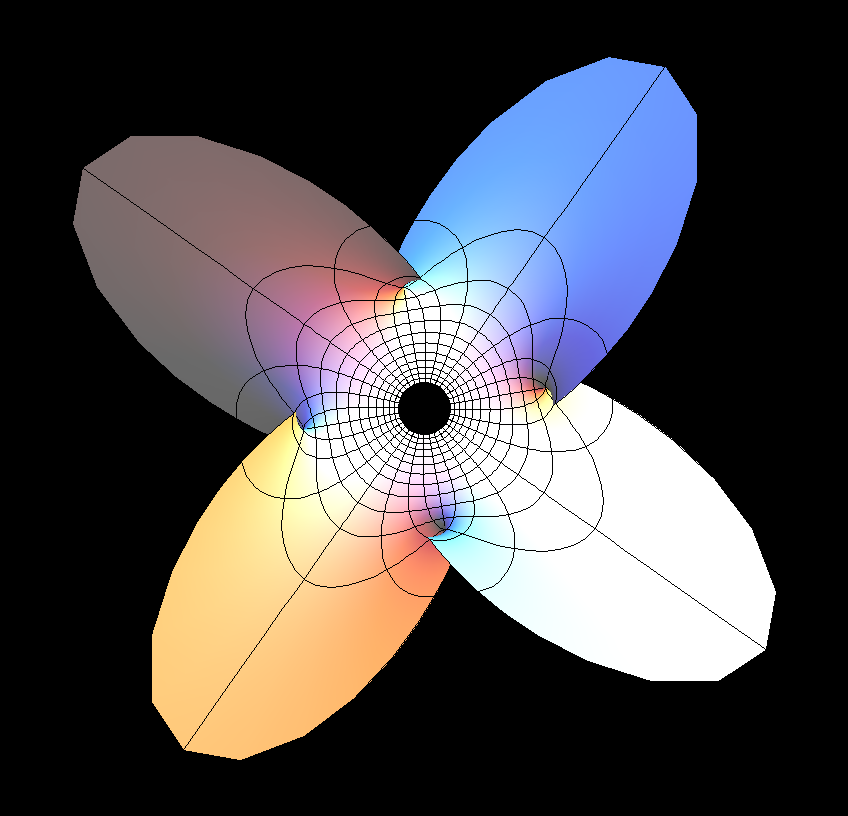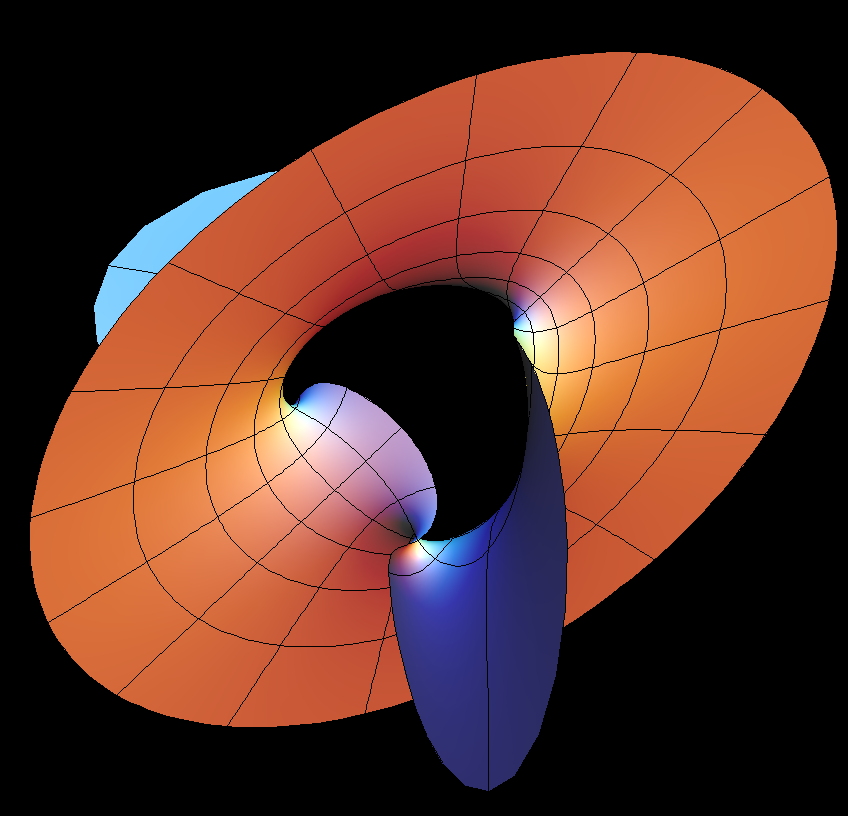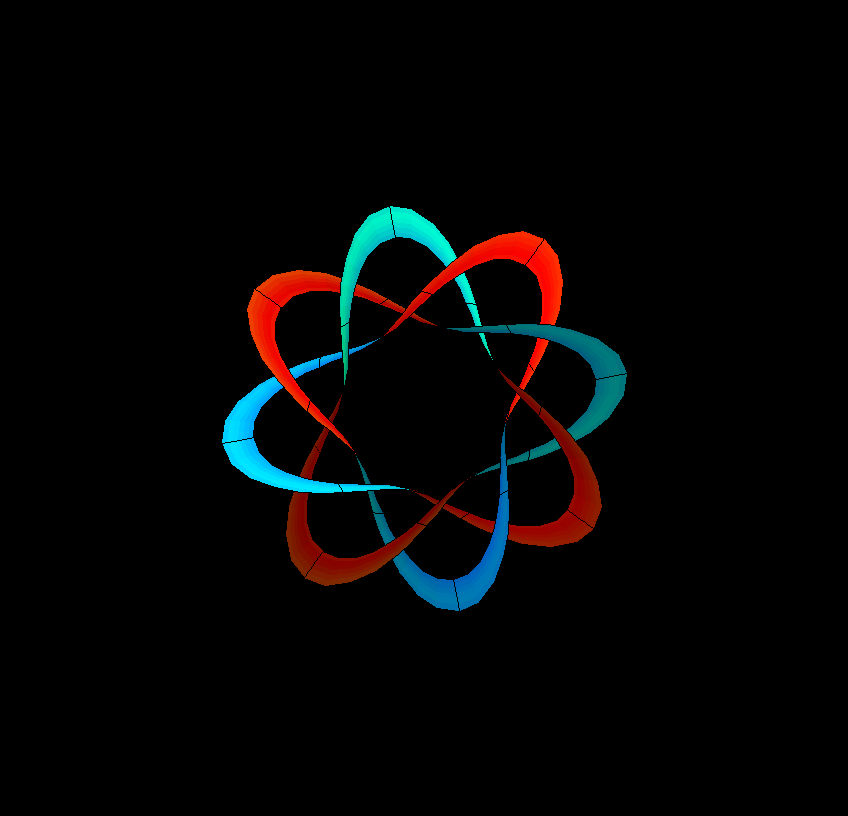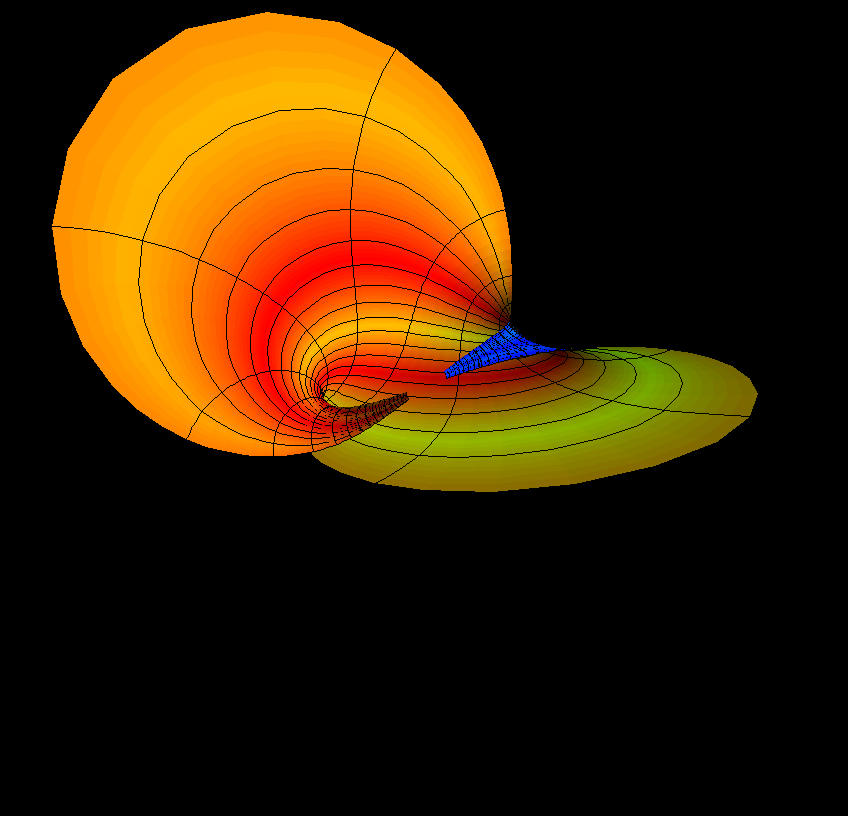# Kusner Surface

On the one hand this minimal surface is so complicated that we can only show images of 4 different smaller parts of the surface. On the other hand, when the surface is described by a map, then the domain is very simple: a sphere with 8 points missing (4 of these so called 'punctures' are on the northern half sphere and 4 on the southern half.A small disk around the north pole is mapped to a propeller shaped part of the surface. Note that 4 straight lines lie on the surface. The lines of which one sees longer parts meet the 4 punctures on the northern half sphere. The 4 short segments extend to lines which pass through the 4 punctures on the southern half sphere.The associate family deformation of a minimal surface is a spectacular phenomenon: the arc length of any curve on the surface remains unchanged and also the Gauss and mean curvature functions of the surface do not change under the deformation.The Planar Enneper minimal surfaces are prototypes of so called “planar ends”. Far out they look like a plane and the puncture is the “infinite point” of that plane. As the plane has come far enough in (meaning “away from infiniy”) it starts to wobble into many different shapes. The 8 planar ends of Kusner's surface all look as in this picture: the outside is planar as for Planar Enneper, but at the inside Kusner's surface crumbles quite differently from Planar Enneper. Notice that one of the parameter lines is one of the straight lines which we already met on the plor cap. (It goes roughly from the left bottom to the right top corner.)A band around the equator is mapped to the 8-3- Torus Knot shown in this animation which widens the band almost until self intersections occur. Again we see portions of the four straight lines on the surface. The orange parts point towards the punctures on one hemisphere, the blue parts towards the punctures on the other hemisphere.One can easily find meridians (from the north to the south pole) which do not come too close to any of the 8 punctures. This animation is again the associate family deformation of a meridian (or longitude) band from the north pole to the southpole. Only one of the surfaces in this family carries straight lines. These lines meet the meridian bands at the poles and therefore force the images of the two poles to coincide: On one image of this animation the meridian band seems to close to a Moebius Strip Moebius strip as on the Inverted Boy Surface . But here only the center line of the meridian band meets exactly.

Pseudo-Code for “Kusner’s Dihedral Symmetry Surfaces”:

Let p = max(Round(ee), 2) and let

```R(z) = u cos(v) I(z) = u sin(v)```

Then `P(z) = R(a(z) V(z)) + (0, 0, aa)`

where `a(z)` is the complex number

`a(z) = 1/ (z^p − z^-p + (2/(p − 1)) sqrt(2p − 1))`

and `V(z)` is the complex vector

`V(z) = (i(z^(p−1) + z^(1−p)), z^(p−1) + z^(1−p), (i (p − 1)/p)(z^p + z^(−p)))`

This is a minimal surface with dihedral symmetry of order 2p if p is odd and 4p if p iseven. The default value of ee is 4. This gives the inversion in the unit sphere of the “Morin Sphere Eversion Midpoint” Willmore surface (see the surface surface menu). On the other hand, when ee = 3 this gives the Inverted Boy Surface.

For full details, see: [R. Kusner, Conformal Geometry and Complete Minimal Surfaces, Bulletin of the AMS, v.17, Number 2, October 1987, pp291--295.]